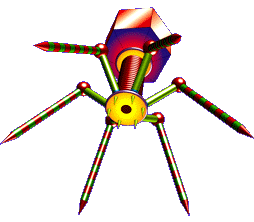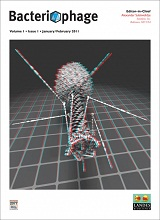# Biology as Poetry: Genetics## Punnett Square

Means of visually probability theory calculations particularly for estimating genotype frequencies among progeny of specific crosses.

The Punnett square is most useful for crosses in which only a single locus is considered since these involve only a 2 × 2 output. To some degree the Punnett square may also be employed where two loci along two alleles at each locus are considered. The resulting 4 × 4 matrix is about as large as may be useful, however.

By three loci, with two alleles at each, the size is already up to 8 × 8, or 64 total combinations. Consequently, for doing genetics calculations above the very basic it is imperative that one learn the basics of probability theory.